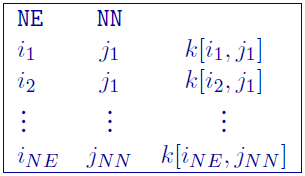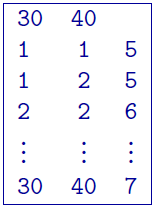# 5.2.3. GIF discrete topography file¶

This file is used specifically with DCIP3D to define the surface topography within a mesh by the integer values of the number of cells from the top of the mesh to the discretized topographic surface. This format is compatible with any type of DC/IP 3D data set. This file is usually created with the make_wdat executable found in the DC/IP 3D inversion package and is required for borehole 3D DC/IP data. The format is:Parameter definitions:

• NN: Number of cells in the north ($$NN$$ from the mesh)

• NE: Number of cells in the north ($$NE$$ from the mesh)

• $$i_n$$: $$n^{th}$$ cell in the easting direction, starting with the west-most cells = 1

• $$j_m$$: $$m^{th}$$ cell in the northing direction, starting with the south-most cells = 1

• $$k[i_n,j_m]$$: Number of cells from top of the mesh to the topography

The lines in this file can be in any order as long as the total number is equal to $$NE \times NN$$. There are no comments allowed in this file. The value of $$k[i,j]$$ indicates the number of cells above the earth’s surface. If the topography reached the top row of cells in the vertical direction, then $$k[i,j] = 0$$. If all values of $$k$$ are zero, the topography would be at the very top of the mesh (i.e., the situation where no topography is given).

## 5.2.3.1. Example:¶

The following is an example of a discrete topography file:In this example the topography at $$(1,2)$$ is higher than at $$(2,2)$$ by one cell thickness. The mesh consists of 30 cells in the easting, 40 cells in the northing, and at least 7 cells in the vertical direction. If the topography is above the mesh, then $$k=0$$.